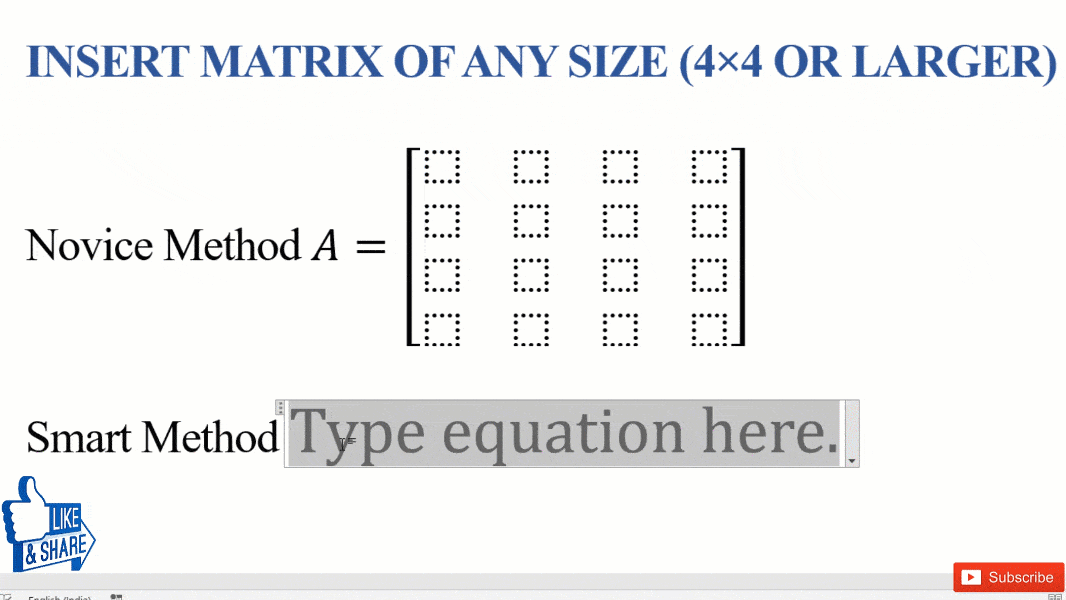# [Shortcut] Make a 4×4 matrix in WordFor a variety of reasons, you may need to make a 4×4 Matrix in Word or even larger Matrix. There are two ways to insert custom size Matrix in Word.

## Two ways to get custom size Matrix in Word

• Normal: Insert 3 x 3 matrix and keep adding additional rows and columns
• Smart: Use shortcut i.e. \matrix(@@@&&&). @ and & are used to represent rows and columns, respectively.

Equation editor method is faster and saves time & effort. However, they are seldom talked about in Microsoft community and forums.

### Normal Method to make 4×4 or large matrix in word

This method is commonly used and involves getting equation editor, inserting 3 x 3 matrix and adding required number of additional rows and columns. Inserting each additional rows/columns involves additional work .Step-by-Step Method to Insert 4×4 matrix or larger matrix in Ms Word

#### Steps to insert 4 x 4 or larger matrix in Word are:

• Get Equation Editor: Navigate to “Symbols Group” in “Insert tab” and click on “Equations
• Inserting 3×3 matrix
• Insert enclosing bracket — (), [] or {}, for matrix, and bring cursor inside the brackets
• Navigate to “Structure Group” in “Design Tab” and click on “Matrix button” and select the 3 x 3 matrix (which is the largest among available)
• Then, Right-click any cell of matrix, and from Insert select “Insert Row (or Columns) Before (or After)” to insert desired number of rows and columns.

### Smart Method to make 4×4 or large matrix in word

This method involves use of Math Autocorrect feature of Ms Word. It’s enable for Ms Word 2007 and above and is activated by default. Means you don’t have to do additional setting to use it.

#### Steps to insert 4 x 4 or larger matrix in Word using equation editor shortcut are

• Get Equation Editor: Use keyboard shortcut “Alt + =”
• Equation Editor Shortcut: Type “[\matrix(@@@&&&)<sp>]<sp>” to get 4 x 4 matrix. Number of @ and & describes size of matrix.

Note:

• Number of @ = Number of rows – 1
• Number of & = Number of columns – 1
• <sp> stands for spacebar

Equation editor shortcut can create a matrix of any size.For e.g. to get 5×5 matrix use \matrix(@@@@&&&&)<sp> and for 4×6 matrix use \matrix(@@@&&&&&)<sp>. This is just one of the matrix shortcut, to learn more shortcut on creating matrix (like identity matrix and more) visit our other useful blog on Matrix. You may also like our blog on complete reference to Equation Editor Shortcut.

For more useful equation editor shortcut on Matrix and more

## Conclusion

To make 4×4 matrix or larger matrix in Word, normal process involves adding 3×3 matrix and then inserting rows and columns. However equation editor shortcut reduces the entire process into typing \matrix(@@@&&&).